Students can go through AP Board 8th Class Maths Notes Chapter 13 Visualizing 3-D in 2-D to understand and remember the concepts easily.

## AP State Board Syllabus 8th Class Maths Notes Chapter 13 Visualizing 3-D in 2-D

→ Polyhedron: Solid objects having flat surfaces.

→ Prism: The polyhedra have top and base as same polygon and other faces are rectangular (parallelogram).

→ Pyramid: Polyhedron which have a polygon as base and a vertex, rest of the faces are triangles. 4 3-D objects could be make by using 2-D nets.→ Euler’s formula for polyhedra : E + 2 = F + V.

→ 3-D Objects made with cubes:
Observe the following solid shapes. Both are formed by arranging four unit cubes. If we observe them from different positions, it seems to be different. But the object is same.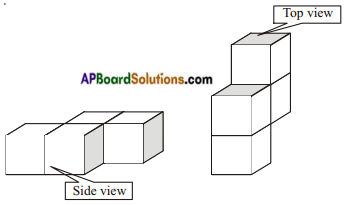→ Faces, Edges and Vertices of 3D-Objects:
Observe the walls, windows, doors, floor, top, corners etc of our living room and tables, boxes etc. Their faces are flat faces. The flat faces meet at its edges. Two or more edges meet at corners. Each of the corner is called vertex. Take a cube and observe it where the faces meet?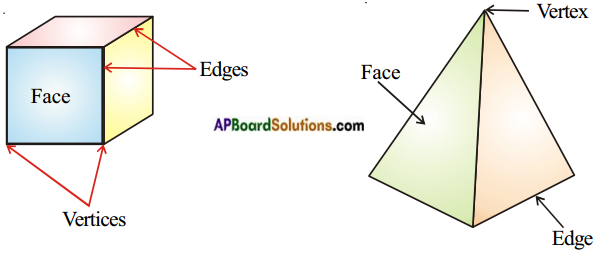→ There are only five regular polyhedra, all of them are complex, often referred as Platonic solids as a tribute to Plato.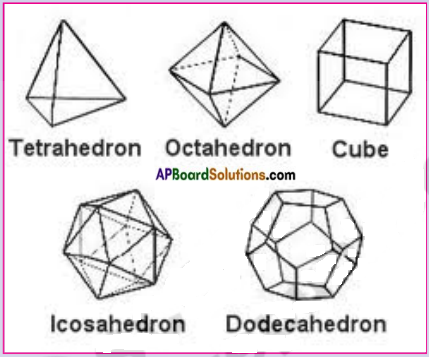Note: Cube is the only polyhedron to completely fill the space.→ Net diagrams of Platonic Solids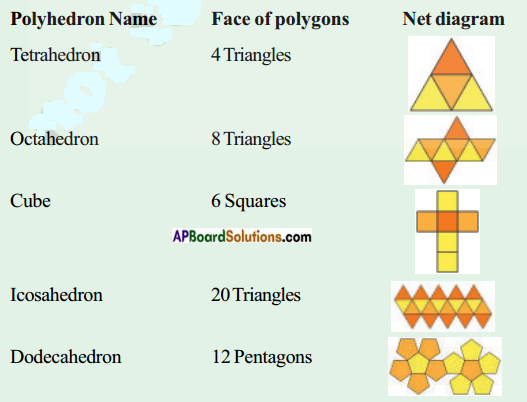→ No. of Edges, Faces and Vertices of Polyhedrons: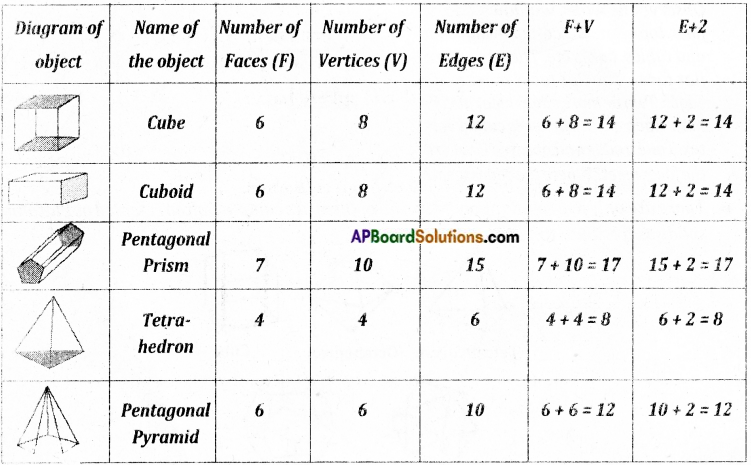By observing the last two columns of the above table, we can conclude that F + V = E + 2 for all polyhedra.